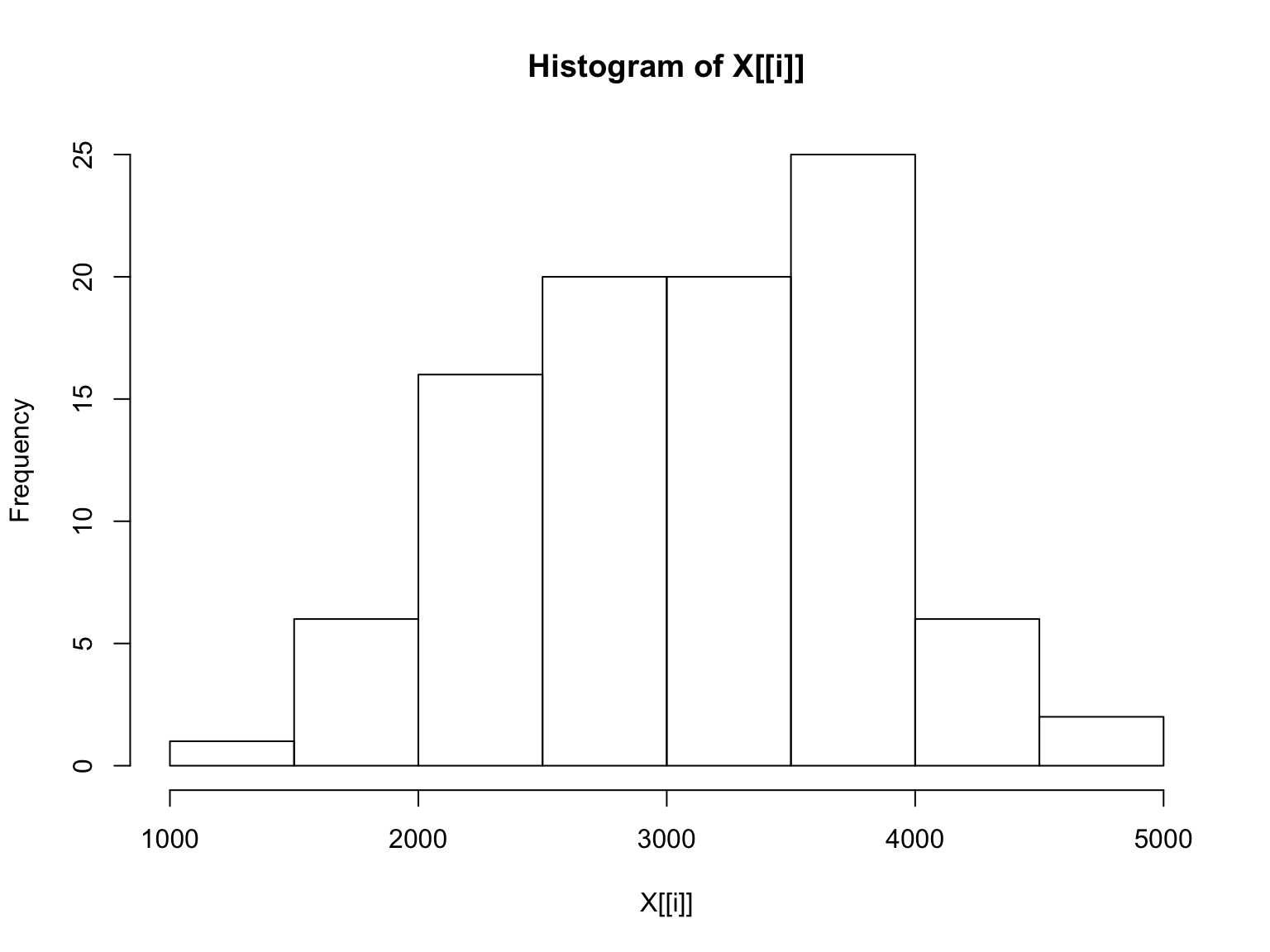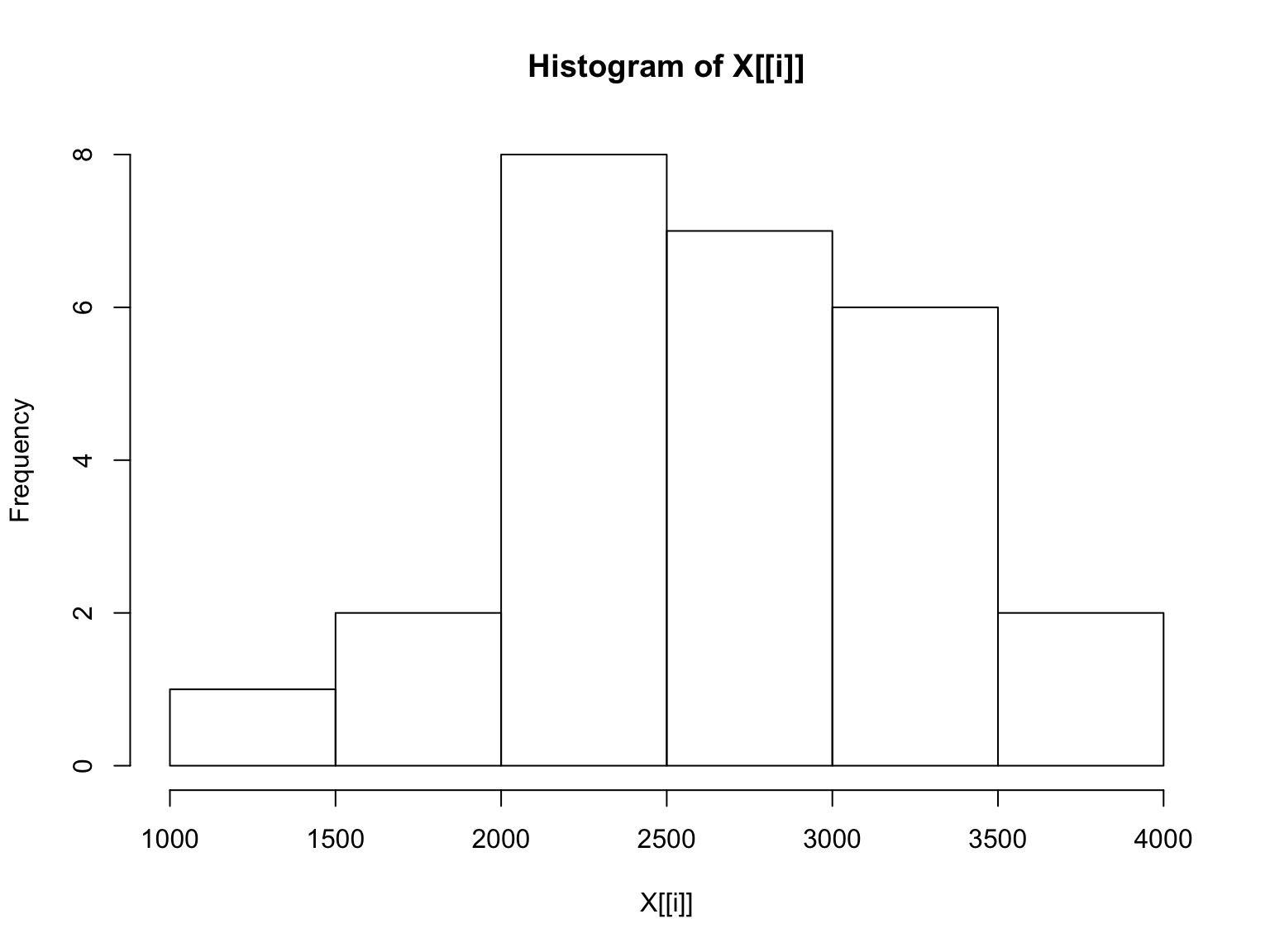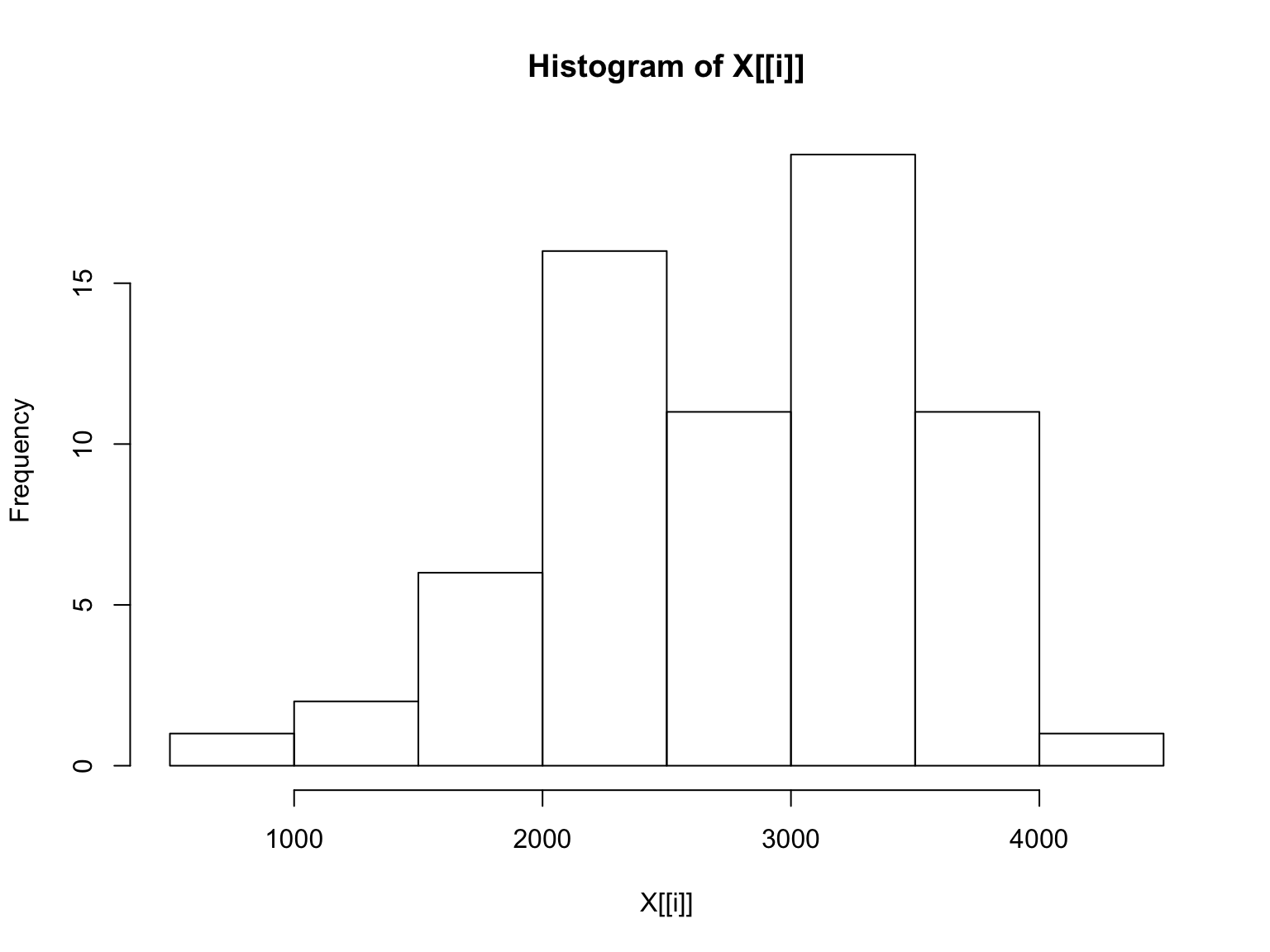# tapply

11/25/2018

## Example of tapply()

First we’ll get some data on birthweights from the MASS package.

This dataframe was created to study the effect of various risk factors on the birthweight of a baby.

Here is the basic data dictionary and a source citation copied from the documentation in the MASS package.

low: indicator of birth weight less than 2.5 kg.

age: mother’s age in years.

lwt: mother’s weight in pounds at last menstrual period.

race: mother’s race (1 = white, 2 = black, 3 = other).

smoke: smoking status during pregnancy.

ptl: number of previous premature labours.

ht: history of hypertension.

ui: presence of uterine irritability.

ftv: number of physician visits during the first trimester.

bwt: birth weight in grams.

Source

Hosmer, D.W. and Lemeshow, S. (1989) Applied Logistic Regression. New York: Wiley

## Get the Data

Once MASS has been downloaded to the computer with the install.packages() command, you should comment out the first line in the chunk below, as I have done.

#install.packages("MASS")
library(MASS)
str(birthwt)
## 'data.frame':    189 obs. of  10 variables:
##  $low : int 0 0 0 0 0 0 0 0 0 0 ... ##$ age  : int  19 33 20 21 18 21 22 17 29 26 ...
##  $lwt : int 182 155 105 108 107 124 118 103 123 113 ... ##$ race : int  2 3 1 1 1 3 1 3 1 1 ...
##  $smoke: int 0 0 1 1 1 0 0 0 1 1 ... ##$ ptl  : int  0 0 0 0 0 0 0 0 0 0 ...
##  $ht : int 0 0 0 0 0 0 0 0 0 0 ... ##$ ui   : int  1 0 0 1 1 0 0 0 0 0 ...
##  $ftv : int 0 3 1 2 0 0 1 1 1 0 ... ##$ bwt  : int  2523 2551 2557 2594 2600 2622 2637 2637 2663 2665 ...

## Exploration

To see if race might be related to birthweight we can use the tapply() function. There are three arguments we need to specify for tapply() to give us useful information.

The first argument is the variable of interest, which is uaually quantitative. In this case it is birthwt$bwt. The second argument is either a categorical variable or a numerical variable with a small number of values. In this case, it is birthwt$race.

The third argument is the name of a function. This function will be applied to subsets of the first argument defined by the values of the second argument. Let’s do this and examine the results.

tapply(birthwt$bwt,birthwt$race,summary)
## $1 ## Min. 1st Qu. Median Mean 3rd Qu. Max. ## 1021 2585 3062 3103 3651 4990 ## ##$2
##    Min. 1st Qu.  Median    Mean 3rd Qu.    Max.
##    1135    2370    2849    2720    3057    3860
##
## $3 ## Min. 1st Qu. Median Mean 3rd Qu. Max. ## 709 2313 2835 2805 3274 4054 We can see the usual summary statistics of birthweigt for the three subsets of our dataframe. We can make the obvious comparisons based on the values of the mean or the median. Race 1 has the highest mean birthweight, etc. Of course, what we’re doing falls far short of formal statistical inference. We’re just “kicking the tires” of our data. We also might want to get other summary statistics, such as the standard deviation, range, or interquartile range not provided by the function summary(). It’s easy to do this by just re-running the code above with the corresponding function names. Let’s do that. print("sd") ##  "sd" tapply(birthwt$bwt,birthwt$race,sd) ## 1 2 3 ## 727.8861 638.6839 722.1944 print("IQR") ##  "IQR" tapply(birthwt$bwt,birthwt$race,IQR) ## 1 2 3 ## 1066.25 686.50 961.00 ## Graphical Results Can we get graphical results? Let’s try to get histograms. tapply(birthwt$bwt,birthwt$race,hist)##$1
## $breaks ##  1000 1500 2000 2500 3000 3500 4000 4500 5000 ## ##$counts
##   1  6 16 20 20 25  6  2
##
## $density ##  2.083333e-05 1.250000e-04 3.333333e-04 4.166667e-04 4.166667e-04 ##  5.208333e-04 1.250000e-04 4.166667e-05 ## ##$mids
##  1250 1750 2250 2750 3250 3750 4250 4750
##
## $xname ##  "X[[i]]" ## ##$equidist
##  TRUE
##
## attr(,"class")
##  "histogram"
##
## $2 ##$breaks
##  1000 1500 2000 2500 3000 3500 4000
##
## $counts ##  1 2 8 7 6 2 ## ##$density
##  7.692308e-05 1.538462e-04 6.153846e-04 5.384615e-04 4.615385e-04
##  1.538462e-04
##
## $mids ##  1250 1750 2250 2750 3250 3750 ## ##$xname
##  "X[[i]]"
##
## $equidist ##  TRUE ## ## attr(,"class") ##  "histogram" ## ##$3
## $breaks ##  500 1000 1500 2000 2500 3000 3500 4000 4500 ## ##$counts
##   1  2  6 16 11 19 11  1
##
## $density ##  2.985075e-05 5.970149e-05 1.791045e-04 4.776119e-04 3.283582e-04 ##  5.671642e-04 3.283582e-04 2.985075e-05 ## ##$mids
##   750 1250 1750 2250 2750 3250 3750 4250
##
## $xname ##  "X[[i]]" ## ##$equidist
##  TRUE
##
## attr(,"class")
##  "histogram"

The labels are very poor, but in this situation, with only a few values of the second argument, we know that the first histogram is for race 1, etc.

## Freedom with the First Argument

Does the first argument have to be quantitative? Let’s try to get a table of the smoke variable for each value of the race variable.

tapply(birthwt$smoke,birthwt$race,table)
## $1 ## ## 0 1 ## 44 52 ## ##$2
##
##  0  1
## 16 10
##
## $3 ## ## 0 1 ## 55 12 Yes, this does work, but there are easier ways to do this, which produce more readable output. The simple base R table() command with two arguments is one way. table(birthwt$race,birthwt$smoke) ## ## 0 1 ## 1 44 52 ## 2 16 10 ## 3 55 12 ## Summary I started out to just clarify the use of tapply(), but I saw some related things, which I felt compelled to discuss, primarily to point out that tapply() is not the best tool for all situations. What I want you to do is apply the usage rules of tapply() to a few examples. ## Exercise 1 Use tapply() to produce summary statistics, the standard deviation, and the IQR, of the variable bwt for the subsets of birthwt defined by the variable smoke. Note that this requires three calls to tapply(). Do this yourself before you look at the answer on the next slide. ## Answer print("Basic Summary") ##  "Basic Summary" tapply(birthwt$bwt,birthwt$smoke,summary) ##$0
##    Min. 1st Qu.  Median    Mean 3rd Qu.    Max.
##    1021    2509    3100    3056    3622    4990
##
## $1 ## Min. 1st Qu. Median Mean 3rd Qu. Max. ## 709 2370 2776 2772 3246 4238 print("Standard Deviation") ##  "Standard Deviation" tapply(birthwt$bwt,birthwt$smoke,sd) ## 0 1 ## 752.6566 659.6349 print("IQR") ##  "IQR" tapply(birthwt$bwt,birthwt$smoke,IQR) ## 0 1 ## 1112.50 875.25 ## Exercise 2 Use tapply() to produce summary statistics, the standard deviation, and the IQR, of the variable ftv (first trimester visits) for the subsets of birthwt defined by the variable low (low birthweight). Note that this requires three calls to tapply(). Do this yourself before you look at the answer on the next slide. ## Answer print("Basic Summary") ##  "Basic Summary" tapply(birthwt$ftv,birthwt$low,summary) ##$0
##    Min. 1st Qu.  Median    Mean 3rd Qu.    Max.
##  0.0000  0.0000  1.0000  0.8385  1.0000  6.0000
##
## $1 ## Min. 1st Qu. Median Mean 3rd Qu. Max. ## 0.0000 0.0000 0.0000 0.6949 1.0000 4.0000 print("Standard Deviation") ##  "Standard Deviation" tapply(birthwt$ftv,birthwt$low,sd) ## 0 1 ## 1.069694 1.038139 print("IQR") ##  "IQR" tapply(birthwt$ftv,birthwt$low,IQR) ## 0 1 ## 1 1 ## Exercise 2 Use tapply() to produce summary statistics, the standard deviation, and the IQR, of the variable age for the subsets of birthwt defined by the variable low. Note that this requires three calls to tapply(). Do this yourself before you look at the answer on the next slide. ## Answer print("Basic Summary") ##  "Basic Summary" tapply(birthwt$age,birthwt$low,summary) ##$0
##    Min. 1st Qu.  Median    Mean 3rd Qu.    Max.
##   14.00   19.00   23.00   23.66   28.00   45.00
##
## $1 ## Min. 1st Qu. Median Mean 3rd Qu. Max. ## 14.00 19.50 22.00 22.31 25.00 34.00 print("Standard Deviation") ##  "Standard Deviation" tapply(birthwt$age,birthwt$low,sd) ## 0 1 ## 5.584522 4.511496 print("IQR") ##  "IQR" tapply(birthwt$age,birthwt\$low,IQR)
##   0   1
## 9.0 5.5